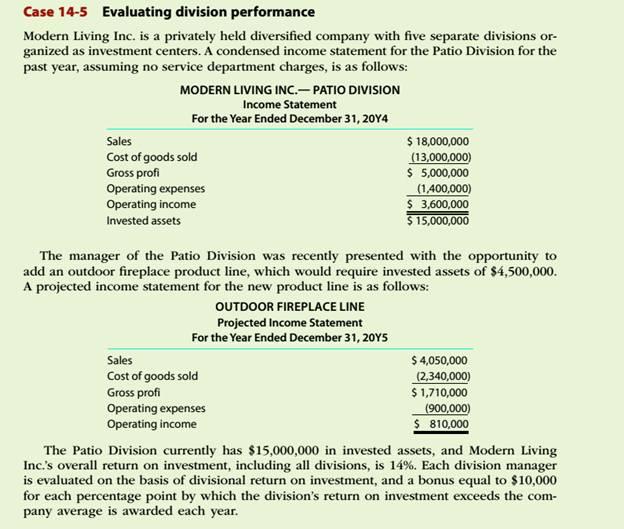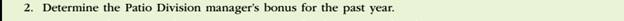Chapter
Section
Textbook ProblemTo determine

Concept Introduction:

Return on investment (ROI):

Return on investment is a profitability ratio that represents the percentage return on the investment made. It is calculated by dividing the Net Income by the Average total assets. The formulas to calculate the ROI are as follows:

ROI = Operating IncomeAverage total assets

ROI (Expanded)= Operating IncomeSales ×SalesAverage total assets

Or

ROI = Profit Margin Ratio ×Asset Turnover ratio

To Calculate:

The manager's bonus for the past year

Explanation

The manager's bonus for the past year is calculated as follows:

 Operating Income (A) $3,600,000 Invested Assets (B)$ 15,000,000

Still sussing out bartleby?

Check out a sample textbook solution.

See a sample solution

The Solution to Your Study Problems

Bartleby provides explanations to thousands of textbook problems written by our experts, many with advanced degrees!

Get Started

ADJUSTING COST OF CAPITAL FOR RISK Ziege Systems is considering the following independent projects for the comi...

Fundamentals of Financial Management, Concise Edition (with Thomson ONE - Business School Edition, 1 term (6 months) Printed Access Card) (MindTap Course List)

Explain why economists usually oppose controls on prices.

Principles of Macroeconomics (MindTap Course List)

List and discuss three key facts about economic fluctuations.

Brief Principles of Macroeconomics (MindTap Course List)

In what ways is economics a science?

Essentials of Economics (MindTap Course List)